# TensorFlow.js - 使用 CNN 识别手写数字

## 您将构建的模型

• 加载数据。
• 定义模型的架构。
• 训练模型并监控其训练时的性能。
• 通过进行一些预测来评估经过训练的模型。

## 您将学到的内容

• TensorFlow.js 语法，用于使用 TensorFlow.js Layers API 创建卷积模型。
• 在 TensorFlow.js 中制定分类任务
• 如何使用 tfjs-vis 库监控浏览器内训练。

## 创建 HTML 网页并添加 JavaScript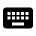将以下代码复制到名为 index.html 的 HTML 文件中

`index.html`

``````<!DOCTYPE html>
<html>
<meta charset="utf-8">
<meta http-equiv="X-UA-Compatible" content="IE=edge">
<meta name="viewport" content="width=device-width, initial-scale=1.0">
<title>TensorFlow.js Tutorial</title>

<!-- Import TensorFlow.js -->
<script src="https://cdn.jsdelivr.net/npm/@tensorflow/tfjs@1.0.0/dist/tf.min.js"></script>
<!-- Import tfjs-vis -->
<script src="https://cdn.jsdelivr.net/npm/@tensorflow/tfjs-vis@1.0.2/dist/tfjs-vis.umd.min.js"></script>

<!-- Import the data file -->
<script src="data.js" type="module"></script>

<!-- Import the main script file -->
<script src="script.js" type="module"></script>

<body>
</body>
</html>
``````

## 为数据和代码创建 JavaScript 文件

1. 在上述 HTML 文件所在的文件夹中，创建一个名为 data.js 的文件，并将此链接中的内容复制到该文件中。
2. 在与第 1 步相同的文件夹中，创建一个名为 script.js 的文件，并将以下代码放入其中。
``````console.log('Hello TensorFlow');
``````

## 开始测试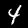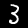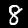• `nextTrainBatch(batchSize)`：从训练集返回随机批次的图片及其标签。
• `nextTestBatch(batchSize)`：从测试集中返回一批图片及其标签

MnistData 类还执行了重排数据和将数据归一化的重要步骤。将以下代码添加到您的 script.js 文件中。

``````import {MnistData} from './data.js';

async function showExamples(data) {
// Create a container in the visor
const surface =
tfvis.visor().surface({ name: 'Input Data Examples', tab: 'Input Data'});

// Get the examples
const examples = data.nextTestBatch(20);
const numExamples = examples.xs.shape;

// Create a canvas element to render each example
for (let i = 0; i < numExamples; i++) {
const imageTensor = tf.tidy(() => {
// Reshape the image to 28x28 px
return examples.xs
.slice([i, 0], [1, examples.xs.shape])
.reshape([28, 28, 1]);
});

const canvas = document.createElement('canvas');
canvas.width = 28;
canvas.height = 28;
canvas.style = 'margin: 4px;';
await tf.browser.toPixels(imageTensor, canvas);
surface.drawArea.appendChild(canvas);

imageTensor.dispose();
}
}

async function run() {
const data = new MnistData();
await showExamples(data);
}

``````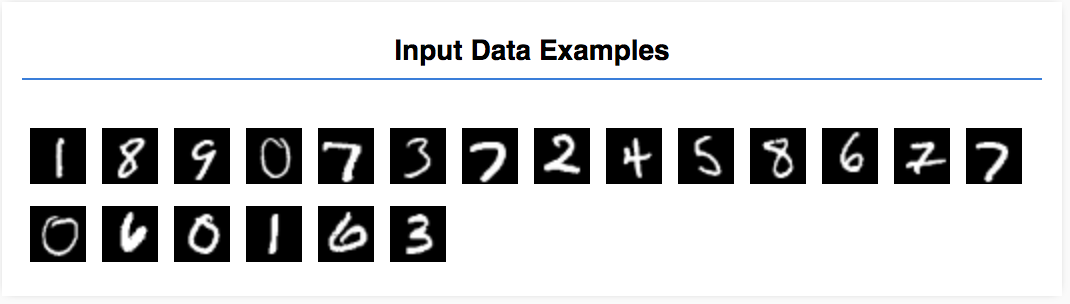将以下函数添加到您的

`script.js` 文件来定义模型架构

``````function getModel() {
const model = tf.sequential();

const IMAGE_WIDTH = 28;
const IMAGE_HEIGHT = 28;
const IMAGE_CHANNELS = 1;

// In the first layer of our convolutional neural network we have
// to specify the input shape. Then we specify some parameters for
// the convolution operation that takes place in this layer.
inputShape: [IMAGE_WIDTH, IMAGE_HEIGHT, IMAGE_CHANNELS],
kernelSize: 5,
filters: 8,
strides: 1,
activation: 'relu',
kernelInitializer: 'varianceScaling'
}));

// The MaxPooling layer acts as a sort of downsampling using max values
// in a region instead of averaging.
model.add(tf.layers.maxPooling2d({poolSize: [2, 2], strides: [2, 2]}));

// Repeat another conv2d + maxPooling stack.
// Note that we have more filters in the convolution.
kernelSize: 5,
filters: 16,
strides: 1,
activation: 'relu',
kernelInitializer: 'varianceScaling'
}));
model.add(tf.layers.maxPooling2d({poolSize: [2, 2], strides: [2, 2]}));

// Now we flatten the output from the 2D filters into a 1D vector to prepare
// it for input into our last layer. This is common practice when feeding
// higher dimensional data to a final classification output layer.

// Our last layer is a dense layer which has 10 output units, one for each
// output class (i.e. 0, 1, 2, 3, 4, 5, 6, 7, 8, 9).
const NUM_OUTPUT_CLASSES = 10;
units: NUM_OUTPUT_CLASSES,
kernelInitializer: 'varianceScaling',
activation: 'softmax'
}));

// Choose an optimizer, loss function and accuracy metric,
// then compile and return the model
model.compile({
optimizer: optimizer,
loss: 'categoricalCrossentropy',
metrics: ['accuracy'],
});

return model;
}
``````

## 卷积

``````model.add(tf.layers.conv2d({
inputShape: [IMAGE_WIDTH, IMAGE_HEIGHT, IMAGE_CHANNELS],
kernelSize: 5,
filters: 8,
strides: 1,
activation: 'relu',
kernelInitializer: 'varianceScaling'
}));
``````

• `inputShape`。将流入模型第一层的数据的形状。在本例中，我们的 MNIST 示例是 28x28 像素的黑白图片。图片数据的规范格式为 `[row, column, depth]`，因此在这里我们需要配置以下形状：`[28, 28, 1]`。各个维度的像素数量为 28 行和 28 列，深度为 1，因为我们的图片只有一个颜色通道。请注意，我们不会在输入形状中指定批次大小。层设计为与批次大小无关，因此在推理期间，您可以传入任何批次大小的张量。
• `kernelSize`。要应用于输入数据的滑动卷积过滤器窗口的尺寸。在此示例中，我们将 `5` 设为 `kernelSize`，以指定方形的 5x5 卷积窗口。
• `filters`。要应用于输入数据的尺寸为 `kernelSize` 的过滤器窗口数量。在此示例中，我们将对数据应用 8 个过滤器。
• `strides`。滑动窗口的“步长”，即每次移动图片时过滤器都会移动多少像素。我们指定步长为 1，表示过滤器将以 1 像素为步长在图片上滑动。
• `activation`。卷积完成后应用于数据的激活函数。在本例中，我们将应用修正线性单元 (ReLU) 函数，这是机器学习模型中非常常见的激活函数。
• `kernelInitializer`。用于随机初始化模型权重的方法，这对于训练动态非常重要。我们在这里不详细介绍初始化，但此处使用的 `VarianceScaling` 通常是一种很好的初始化程序选择

## 展平数据表示法

``````model.add(tf.layers.flatten());
``````

## 计算我们的最终概率分布

``````const NUM_OUTPUT_CLASSES = 10;
units: NUM_OUTPUT_CLASSES,
kernelInitializer: 'varianceScaling',
activation: 'softmax'
}));
``````

## 选择优化器和损失函数

``````const optimizer = tf.train.adam();
model.compile({
optimizer: optimizer,
loss: 'categoricalCrossentropy',
metrics: ['accuracy'],
});
``````

 索引 0 1 次 2 3 4 5 6 7 8 9 真实标签 0 0 0 0 0 0 0 1 0 0 预测 0.1 0.01 0.01 0.01 0.2 0.01 0.01 0.6 0.03 0.02将以下函数复制到您的 script.js 文件中。

``````async function train(model, data) {
const metrics = ['loss', 'val_loss', 'acc', 'val_acc'];
const container = {
name: 'Model Training', tab: 'Model', styles: { height: '1000px' }
};
const fitCallbacks = tfvis.show.fitCallbacks(container, metrics);

const BATCH_SIZE = 512;
const TRAIN_DATA_SIZE = 5500;
const TEST_DATA_SIZE = 1000;

const [trainXs, trainYs] = tf.tidy(() => {
const d = data.nextTrainBatch(TRAIN_DATA_SIZE);
return [
d.xs.reshape([TRAIN_DATA_SIZE, 28, 28, 1]),
d.labels
];
});

const [testXs, testYs] = tf.tidy(() => {
const d = data.nextTestBatch(TEST_DATA_SIZE);
return [
d.xs.reshape([TEST_DATA_SIZE, 28, 28, 1]),
d.labels
];
});

return model.fit(trainXs, trainYs, {
batchSize: BATCH_SIZE,
validationData: [testXs, testYs],
epochs: 10,
shuffle: true,
callbacks: fitCallbacks
});
}
``````然后将以下代码添加到您的

`run` 函数。

``````const model = getModel();
tfvis.show.modelSummary({name: 'Model Architecture', tab: 'Model'}, model);

await train(model, data);
``````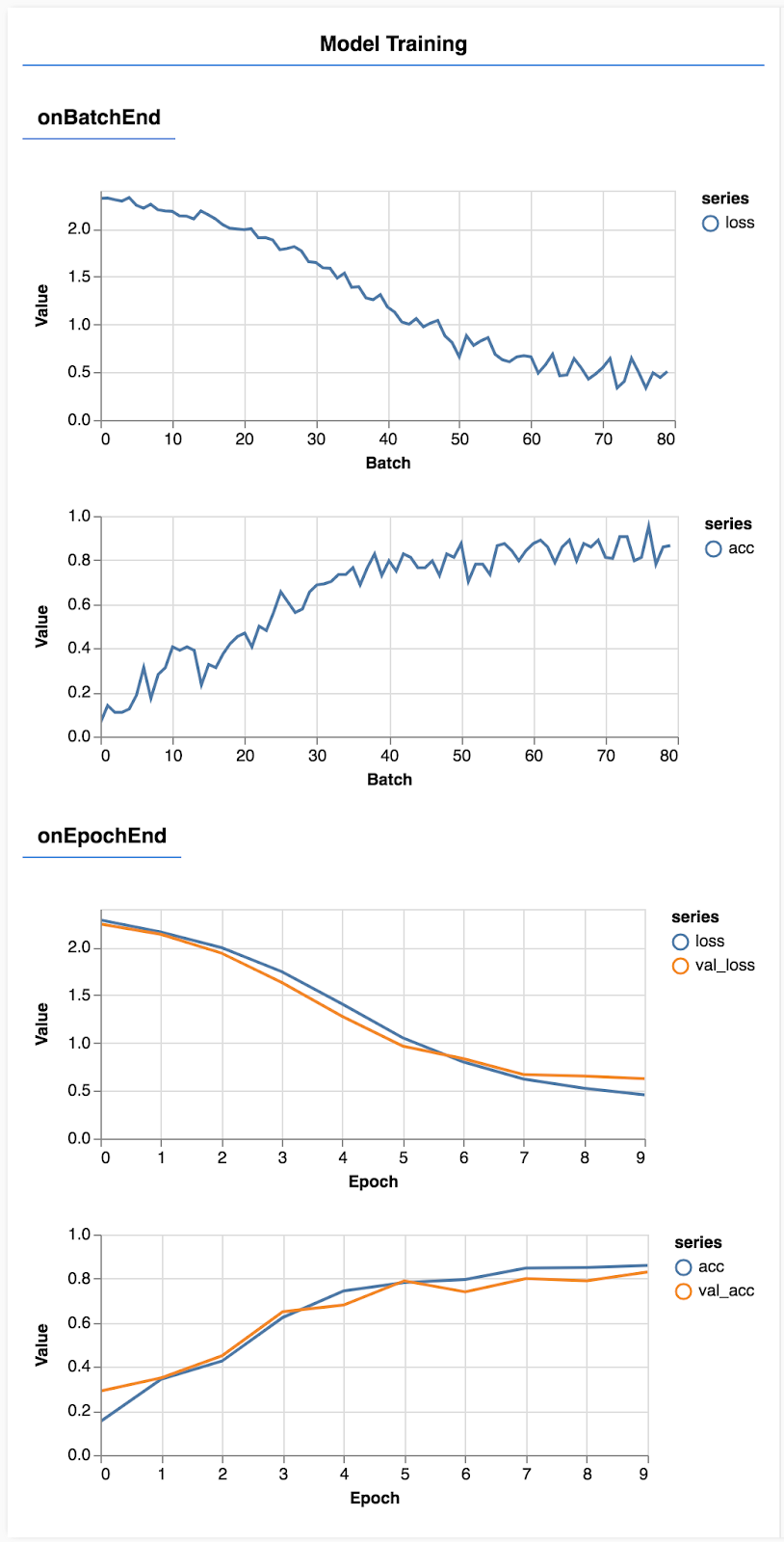## 监控指标

``````const metrics = ['loss', 'val_loss', 'acc', 'val_acc'];
``````

## 准备作为张量的数据

``````const BATCH_SIZE = 512;
const TRAIN_DATA_SIZE = 5500;
const TEST_DATA_SIZE = 1000;

const [trainXs, trainYs] = tf.tidy(() => {
const d = data.nextTrainBatch(TRAIN_DATA_SIZE);
return [
d.xs.reshape([TRAIN_DATA_SIZE, 28, 28, 1]),
d.labels
];
});

const [testXs, testYs] = tf.tidy(() => {
const d = data.nextTestBatch(TEST_DATA_SIZE);
return [
d.xs.reshape([TEST_DATA_SIZE, 28, 28, 1]),
d.labels
];
});
``````

``````return model.fit(trainXs, trainYs, {
batchSize: BATCH_SIZE,
validationData: [testXs, testYs],
epochs: 10,
shuffle: true,
callbacks: fitCallbacks
});
``````

tfjs-vis 中有一些方法可以帮助您实现上述目标。将以下代码添加到 script.js 文件的底部

``````const classNames = ['Zero', 'One', 'Two', 'Three', 'Four', 'Five', 'Six', 'Seven', 'Eight', 'Nine'];

function doPrediction(model, data, testDataSize = 500) {
const IMAGE_WIDTH = 28;
const IMAGE_HEIGHT = 28;
const testData = data.nextTestBatch(testDataSize);
const testxs = testData.xs.reshape([testDataSize, IMAGE_WIDTH, IMAGE_HEIGHT, 1]);
const labels = testData.labels.argMax(-1);
const preds = model.predict(testxs).argMax(-1);

testxs.dispose();
return [preds, labels];
}

async function showAccuracy(model, data) {
const [preds, labels] = doPrediction(model, data);
const classAccuracy = await tfvis.metrics.perClassAccuracy(labels, preds);
const container = {name: 'Accuracy', tab: 'Evaluation'};
tfvis.show.perClassAccuracy(container, classAccuracy, classNames);

labels.dispose();
}

async function showConfusion(model, data) {
const [preds, labels] = doPrediction(model, data);
const confusionMatrix = await tfvis.metrics.confusionMatrix(labels, preds);
const container = {name: 'Confusion Matrix', tab: 'Evaluation'};
tfvis.render.confusionMatrix(container, {values: confusionMatrix, tickLabels: classNames});

labels.dispose();
}

``````

• 进行预测。
• 计算准确率指标。
• 显示指标

## 做出预测

``````function doPrediction(model, data, testDataSize = 500) {
const IMAGE_WIDTH = 28;
const IMAGE_HEIGHT = 28;
const testData = data.nextTestBatch(testDataSize);
const testxs = testData.xs.reshape([testDataSize, IMAGE_WIDTH, IMAGE_HEIGHT, 1]);
const labels = testData.labels.argMax(-1);
const preds = model.predict(testxs).argMax(-1);

testxs.dispose();
return [preds, labels];
}
``````

## 显示每个类的准确率

``````async function showAccuracy() {
const [preds, labels] = doPrediction();
const classAccuracy = await tfvis.metrics.perClassAccuracy(labels, preds);
const container = { name: 'Accuracy', tab: 'Evaluation' };
tfvis.show.perClassAccuracy(container, classAccuracy, classNames);

labels.dispose();
}
``````

## 显示混淆矩阵

``````async function showConfusion() {
const [preds, labels] = doPrediction();
const confusionMatrix = await tfvis.metrics.confusionMatrix(labels, preds);
const container = { name: 'Confusion Matrix', tab: 'Evaluation' };
tfvis.render.confusionMatrix(container, {values: confusionMatrix, tickLabels: classNames});

labels.dispose();
}
``````

## 显示评估将以下代码添加到运行函数的底部，可显示评估。

``````await showAccuracy(model, data);
await showConfusion(model, data);
``````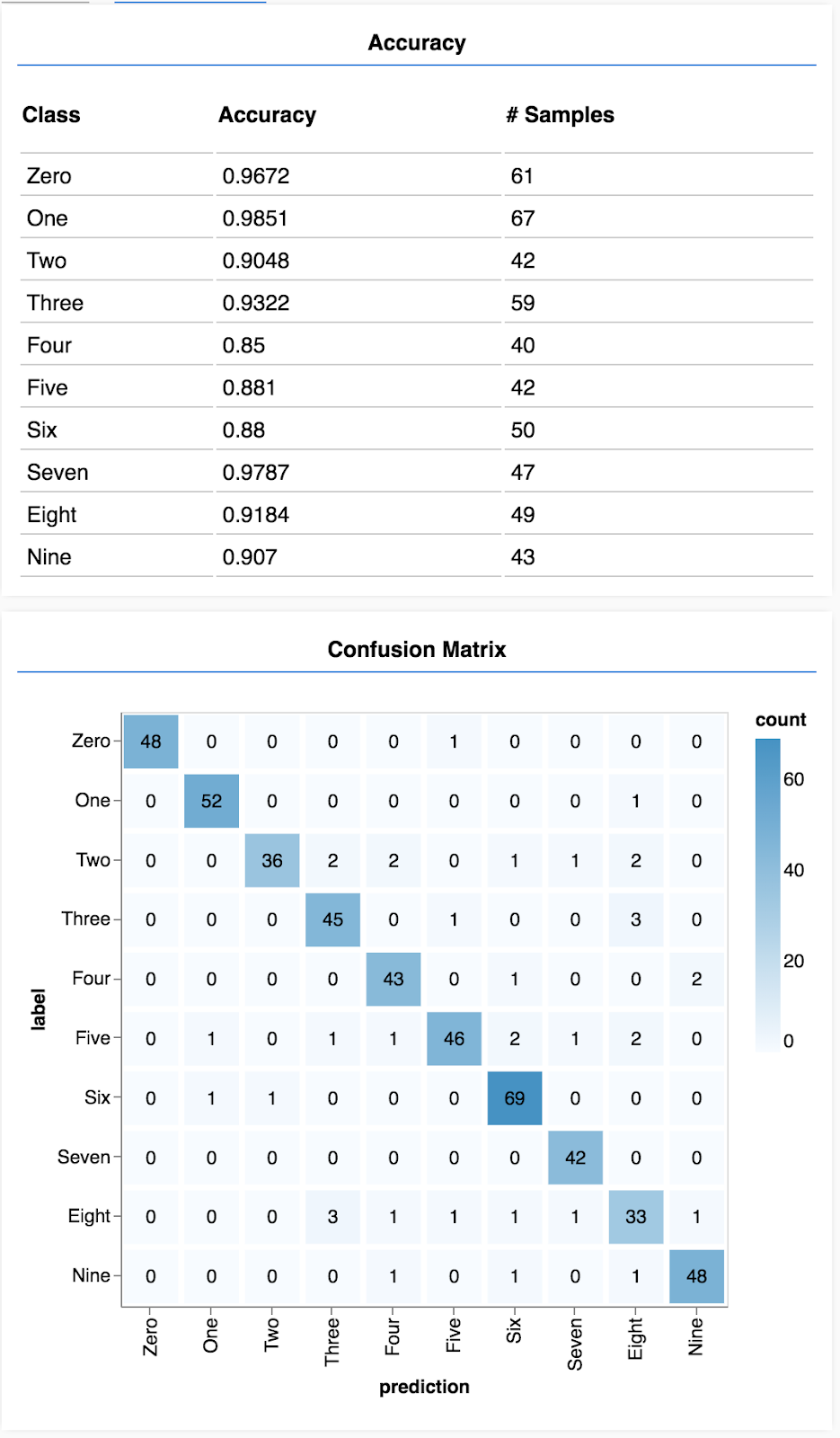• 标签的常见表示法包括对类别进行独热编码

• 将模型在训练期间从未见过并且您可以用于评估模型的一些数据单独保存会很有帮助。这称为验证集。

• 事实证明，卷积模型很适合处理图片任务。
• 分类问题通常使用分类交叉熵作为其损失函数。
• 监控训练，看看损失是否减少，准确率是否上升。

• 确定一些方式，在模型经过训练后对其进行评估，以了解其在处理您要解决的最初问题时表现如何。
• 每个类准确率和混淆矩阵可以为您提供比整体准确率更详细的模型性能。
[{ "type": "thumb-down", "id": "missingTheInformationINeed", "label":"没有我需要的信息" },{ "type": "thumb-down", "id": "tooComplicatedTooManySteps", "label":"太复杂/步骤太多" },{ "type": "thumb-down", "id": "outOfDate", "label":"内容需要更新" },{ "type": "thumb-down", "id": "translationIssue", "label":"翻译问题" },{ "type": "thumb-down", "id": "samplesCodeIssue", "label":"示例/代码问题" },{ "type": "thumb-down", "id": "otherDown", "label":"其他" }]
[{ "type": "thumb-up", "id": "easyToUnderstand", "label":"易于理解" },{ "type": "thumb-up", "id": "solvedMyProblem", "label":"解决了我的问题" },{ "type": "thumb-up", "id": "otherUp", "label":"其他" }]